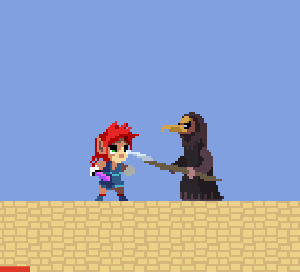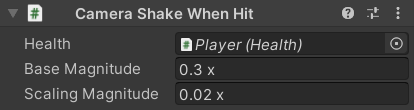﻿ Platformer Game Kit - Camera Shake

# Camera ShakeThe Main Camera prefab has a `CameraShake` component to make it shake in response to certain events based on the GDC talk:

Currently, the only event that causes it is the Player getting hit, which is implemented by the `CameraShakeWhenHit` component attached to the Player prefab, but any other script could easily add to the `CameraShake.Instance.Magnitude`.

# Fields

As explained in the video above, shaking the X and Y Position and the Z Rotation works well for a 2D platformer:The fields have tooltips explaining what they do and Units Attributes where appropriate (unfortunately, they don't work on `Vector3` fields so the tooltips need to specify their units):

``````public sealed class CameraShake : MonoBehaviour
{
[SerializeField]
[Tooltip("The maximum distance the shake can move the camera on each axis (in meters)")]
private Vector3 _PositionStrength;
public ref Vector3 PositionStrength => ref _PositionStrength;

[SerializeField]
[Tooltip("The maximum angle the shake can rotate the camera on each axis (in degrees)")]
private Vector3 _RotationStrength;
public ref Vector3 RotationStrength => ref _RotationStrength;

[SerializeField, Multiplier]
[Tooltip("The rate at which the shake values change")]
private float _Frequency = 6;
public ref float Frequency => ref _Frequency;

[SerializeField, Units(" ^")]
[Tooltip("The current magnitude is raised to this power to calculate the actual shake magnitude")]
private float _MagnitudeExponent = 1;
public ref float MagnitudeExponent => ref _MagnitudeExponent;

[SerializeField, Units(" /s")]
[Tooltip("The rate at which the magnitude decreases")]
private float _Damping = 1.5f;
public ref float Damping => ref _Damping;
``````

The `OnValidate` method ensures that all fields have reasonable values and disables this component (unless it is currently in use, to prevent it from cancelling the shake if you modify its fields in Play Mode):

``````#if UNITY_EDITOR
private void OnValidate()
{
PlatformerUtilities.NotNegative(ref _Frequency);
_MagnitudeExponent = Mathf.Clamp(_MagnitudeExponent, 0.2f, 5f);
PlatformerUtilities.NotNegative(ref _Damping);

if (_Magnitude <= 0)
enabled = false;
}
#endif
``````

The `Magnitude` property is a normalized value which determines how much shake is currently being applied:

• `1` uses the full `_PositionStrength` and `_RotationStrength`.
• `0` turns off the shake.
• The `Magnitude` is constantly decreased by the `_Damping` value.
``````    private float _Magnitude;

public float Magnitude
{
get => _Magnitude;
set
{
_Magnitude = Mathf.Clamp01(value);
enabled = value > 0;
}
}

private void Update()
{
_Magnitude -= _Damping * Time.deltaTime;
if (_Magnitude <= 0)
{
enabled = false;
return;
}
...
``````

It has a static `Instance` property which it assigns itself to on startup so that other scripts (such as the Camera Shake On Hit component) can access it:

``````    public static CameraShake Instance { get; private set; }

private void Awake()
{
Debug.Assert(Instance == null, \$"Another {nameof(CameraShake)} instance already exists", this);
Instance = this;
...
}
``````

Since the `Transform` is used frequently, it is stored in a field on startup to improve performance:

``````    private Transform _Transform;

private void Awake()
{
...
_Transform = transform;
}
``````

The amount of shake applied each frame is stored in the `_PositionOffset` and `_RotationOffset` fields so that on the next frame the previous shake values can be subtracted from the `Transform` before new values are applied. This allows the shake to always be a controlled offset from wherever the camera is supposed to be rather than building upon itself every frame.

``````    private Vector3 _PositionOffset;
private Vector3 _RotationOffset;

private void LateUpdate()
{
...

_Transform.position -= _PositionOffset;
_Transform.eulerAngles -= _RotationOffset;

// ... Calculate new shake values.

_Transform.position += _PositionOffset;
_Transform.eulerAngles += _RotationOffset;
}
``````

The actual `magnitude` of the shake is calculated based on the linearly decreasing `Magnitude` raised to the power of the `_MagnitudeExponent` to improve the way the shake fades out. The reasoning behind this is explained in the video at the top of the page.

``````    private void LateUpdate()
{
...

var magnitude = Mathf.Pow(_Magnitude, _MagnitudeExponent);

var time = _Frequency * Time.timeSinceLevelLoad;

_PositionOffset.x = Noise(_PositionStrength.x, magnitude, 0, time);
_PositionOffset.y = Noise(_PositionStrength.y, magnitude, 1, time);
_PositionOffset.z = Noise(_PositionStrength.z, magnitude, 2, time);
_RotationOffset.x = Noise(_RotationStrength.x, magnitude, 3, time);
_RotationOffset.y = Noise(_RotationStrength.y, magnitude, 4, time);
_RotationOffset.z = Noise(_RotationStrength.z, magnitude, 5, time);

...
}
``````

The actual noise calculation is based on Unity's `Mathf.PerlinNoise` function with a simple check to skip the calculation if the `baseStrength` is `0`:

``````    private static float Noise(float baseStrength, float magnitude, float x, float y) =>
baseStrength == 0 ?
0 :// Avoid the actual Noise lookup for values we aren't using.
baseStrength * magnitude * Noise(x, y);

private static float Noise(float x, float y) => Mathf.PerlinNoise(x, y) * 2 - 1;
``````

If this script is disabled (or destroyed), it undoes its offsets and clears the `Magnitude`:

``````    private void OnDisable()
{
_Transform.position -= _PositionOffset;
_Transform.eulerAngles -= _RotationOffset;

_Magnitude = 0;
_PositionOffset = _RotationOffset = default;
}
}
``````# When Hit

The Player prefab has a `CameraShakeWhenHit` component which registers a callback to their `Health.OnHitReceived` prefab to add to the `CameraShake.Magnitude`. There isn't really anything else in the script that needs explaining.

``````public sealed class CameraShakeWhenHit : MonoBehaviour
{
[SerializeField]
private Health _Health;
public Health Health => _Health;

[SerializeField, Multiplier]
[Tooltip("The shake magnitude to apply regardless of damage taken")]
private float _BaseMagnitude = 0.3f;
public ref float BaseMagnitude => ref _BaseMagnitude;

[SerializeField, Multiplier]
[Tooltip("The additional shake magnitude which is multiplied by the damage taken")]
private float _ScalingMagnitude = 0.02f;
public ref float ScalingMagnitude => ref _ScalingMagnitude;

#if UNITY_EDITOR
private void OnValidate()
{
gameObject.GetComponentInParentOrChildren(ref _Health);
PlatformerUtilities.NotNegative(ref _BaseMagnitude);
PlatformerUtilities.NotNegative(ref _ScalingMagnitude);
}
#endif

private void Awake()
{